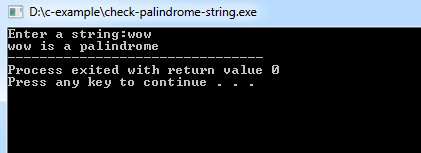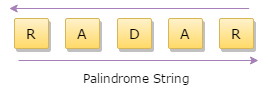## C Programming Examples Tutorial Index

A palindrome is a string, which is the same when read in both forward and backward ways. Identifying such unique strings has applications in data analysis, algorithms, and more. This tutorial guides you through writing a C program to determine if a given string is a palindrome.

Examples of Palindromes:

## How to Write a C Program for Palindrome String Check

Here's a concise, well-commented C code snippet that effectively identifies whether a given string is a palindrome or not.

Example:

``````#include <stdio.h>
#include <string.h>

int main() {
char string1;
int i, length;
int flag = 0;

// Prompt the user for input
printf("Enter a string: ");
scanf("%s", string1);

// Calculate the string length
length = strlen(string1);

// Compare characters from the start and end of the string
// and stop if a mismatch is found or the middle of the string is reached.
for (i = 0; i < length / 2; i++) {
if (string1[i] != string1[length - i - 1]) {
flag = 1;
break;
}
}

// Output the result
if (flag) {
printf("%s is not a palindrome\n", string1);
} else {
printf("%s is a palindrome\n", string1);
}

return 0;
}``````

Program Output:Detailed Explanation:

The above program compares the characters of the string from the beginning and end and stops if a mismatch is found or the middle of the string is reached. If all of the characters match, then the string is a palindrome.

Consider the string "radar". Its character comparison would be as follows:

---------------------------
index: 0 1 2 3 4

value: r a d a r
---------------------------To compare it with the reverse of itself, the following logic is used:

1. The 0th character in the char array, string1, is the same as the 4th character in the same string.
2. 1st character is the same as 3rd character.
3. 2nd character is the same as 2nd character.
4. . . . .
5. ith character is the same as the 'length-i-1'th character.
6. If any of the above conditions fails, the flag is set to true(1), implying that the string is not a palindrome.
7. By default, the value of the flag is false(0). Hence, the string is a palindrome if all the conditions are satisfied.